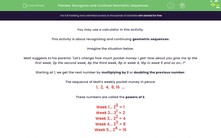# Recognise and Continue Geometric Sequences

In this worksheet, students will recognise and continue geometric sequences.Key stage:  KS 3

Curriculum topic:   Algebra

Curriculum subtopic:   Use Geometric Sequences

Difficulty level:#### Worksheet Overview

You may use a calculator in this activity.

This activity is about recognising and continuing geometric sequences.

Imagine the situation below.

Matt suggests to his parents: "Let's change how much pocket money I get! How about you give me 1p the first week, 2p the second week, 4p the third week, 8p in week 4, 16p in week 5 and so on...?"

Starting at 1, we get the next number by multiplying by 2 or doubling the previous number.

The sequence of Matt's weekly pocket money in pence:

1,  2,  4,  8, 16  ...

These numbers are called the powers of 2.

Week 1... 20 = 1

Week 2... 21 = 2

Week 3... 22 = 4

Week 4... 23 = 8

Week 5... 24 = 16

The 'powers of 2' are an example of a geometric sequence, where each term after the first is found by multiplying the previous term by a fixed number, called the common ratio.

The common ratio here is 2.Let's look at a different geometric sequence!

## 1,  3,  9,  27, ....

What is the common ratio and what is the next term?

Method

To find the common ratio (which means, what does the sequence go up in), we can divide any term by its previous one.

For example 3 ÷ 1 = 3

or we could use 9 ÷ 3 = 3

or the 4th term,  27 ÷ 9 = 3

All of them give us a common ratio of 3

That means to get the next term we multiply the previous term by 3 and the rule increases by powers of 3:

4th term is 27

5th term = 27 x 3 = 81

1st Term ... 30 = 1

2nd Term ... 31 = 3

3rd Term ... 32 = 9

4th Term ... 33 = 27

5th Term... 34 = 81

Notice that the power is one less than the term, ie, the 5th term is the power of 4, or the 3rd term is the power of 2.Let's do some practise!

### What is EdPlace?

We're your National Curriculum aligned online education content provider helping each child succeed in English, maths and science from year 1 to GCSE. With an EdPlace account you’ll be able to track and measure progress, helping each child achieve their best. We build confidence and attainment by personalising each child’s learning at a level that suits them.

Get started